# Electricity Circuits Simple Circuits Series Circuits If you

• Slides: 39Electricity CircuitsSimple Circuits Series Circuits If you connect three ammeters in the circuit, as shown in the figure, they all will show the same current A circuit in which all current travels through each device is called a series circuitSimple Circuits Series Circuits If the current is the same throughout the circuit, what is used by the lamp to produce thermal and light energy? Recall that power, the rate at which electric energy is converted, is represented by P = IV Thus, if there is a potential difference, or voltage drop, across the lamp, then electric energy is being converted into another formSimple Circuits Series Circuits The resistance of the lamp is defined as R = V/I Thus, the potential difference, also called the voltage drop, is V = IR In an electric circuit, the increase in voltage provided by the generator or other energy source, Vsource, is equal to the sum of voltage drops across lamps A and B, and is represented by the following equation: Vsource = VA + VB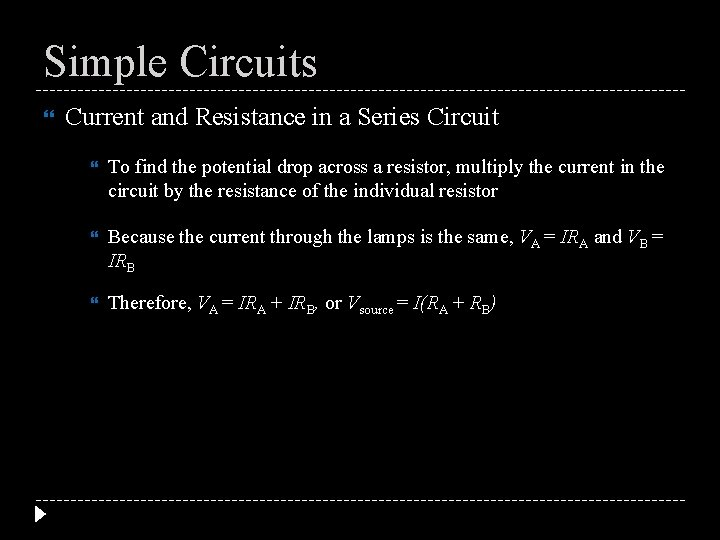Simple Circuits Current and Resistance in a Series Circuit To find the potential drop across a resistor, multiply the current in the circuit by the resistance of the individual resistor Because the current through the lamps is the same, VA = IRA and VB = IRB Therefore, VA = IRA + IRB, or Vsource = I(RA + RB)Simple Circuits Current and Resistance in a Series Circuit The current through the circuit is represented by the following equation: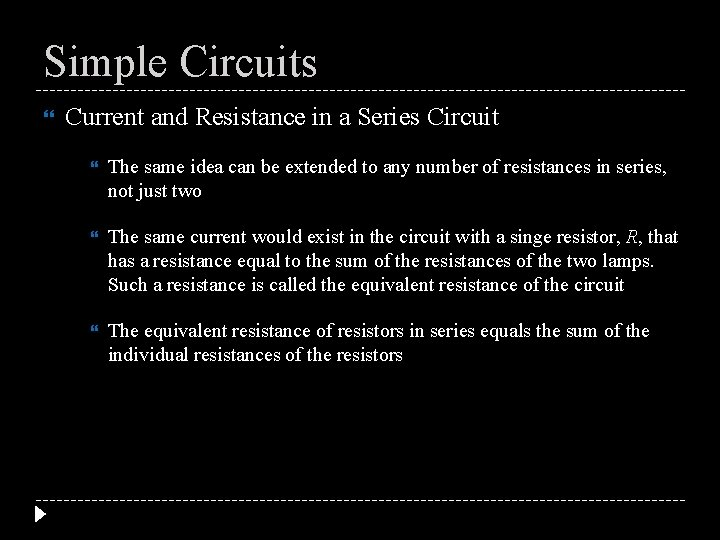Simple Circuits Current and Resistance in a Series Circuit The same idea can be extended to any number of resistances in series, not just two The same current would exist in the circuit with a singe resistor, R, that has a resistance equal to the sum of the resistances of the two lamps. Such a resistance is called the equivalent resistance of the circuit The equivalent resistance of resistors in series equals the sum of the individual resistances of the resistorsSimple Circuits Current and Resistance in a Series Circuit For resistors in series, the equivalent resistance is the sum of all the individual resistances, as expressed by the following equation: Equivalent Resistance for Resistors in Series R = R A + RB + …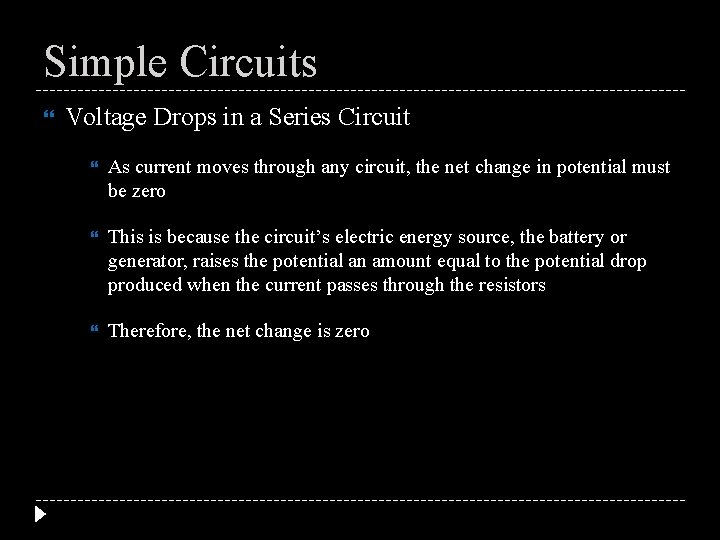Simple Circuits Voltage Drops in a Series Circuit As current moves through any circuit, the net change in potential must be zero This is because the circuit’s electric energy source, the battery or generator, raises the potential an amount equal to the potential drop produced when the current passes through the resistors Therefore, the net change is zero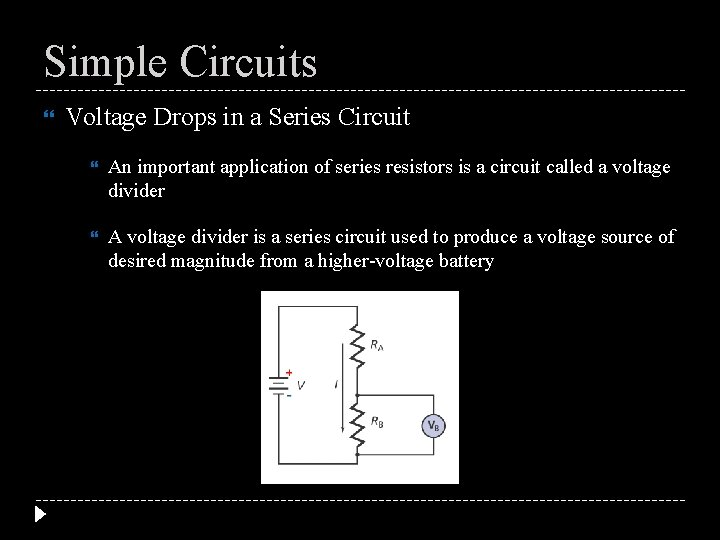Simple Circuits Voltage Drops in a Series Circuit An important application of series resistors is a circuit called a voltage divider A voltage divider is a series circuit used to produce a voltage source of desired magnitude from a higher-voltage battery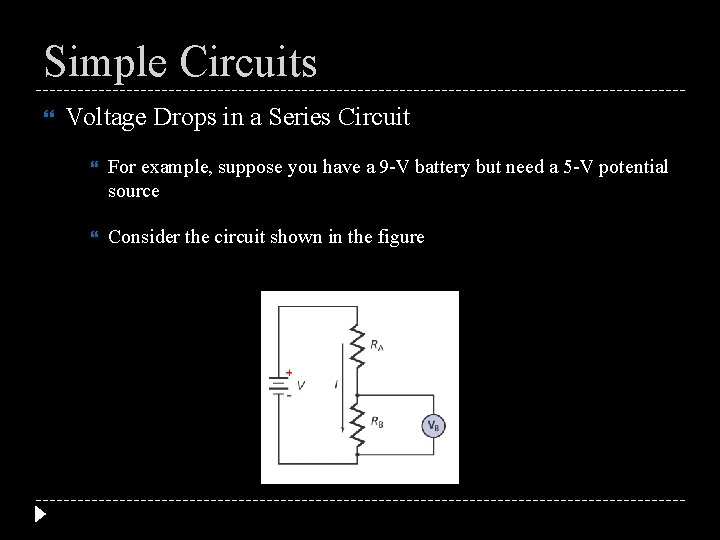Simple Circuits Voltage Drops in a Series Circuit For example, suppose you have a 9 -V battery but need a 5 -V potential source Consider the circuit shown in the figureSimple Circuits Voltage Drops in a Series Circuit Two resistors, RA and RB, are connected in series across a battery of magnitude V The equivalent resistance of the circuit is R = RA + RB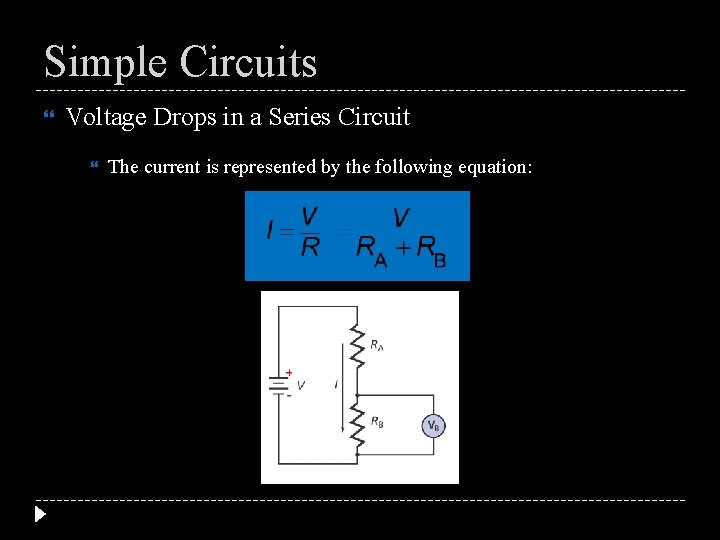Simple Circuits Voltage Drops in a Series Circuit The current is represented by the following equation:Simple Circuits Voltage Drops in a Series Circuit The desired voltage, 5 V, is the voltage drop, VB, across resistor RB: VB = IRB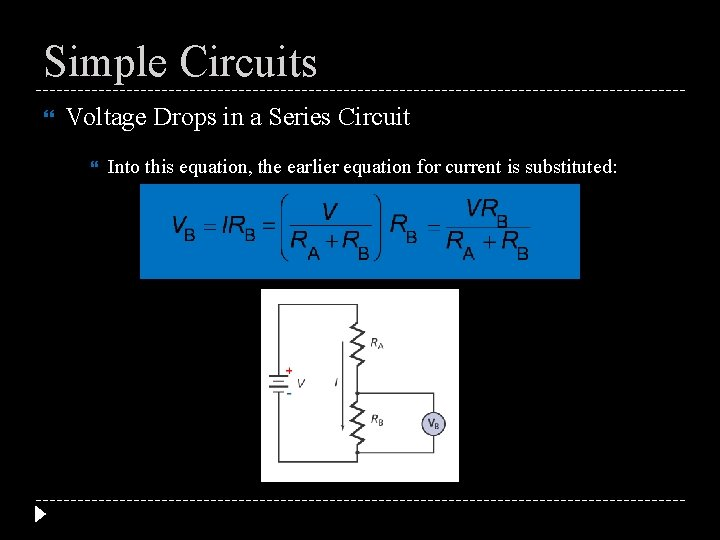Simple Circuits Voltage Drops in a Series Circuit Into this equation, the earlier equation for current is substituted: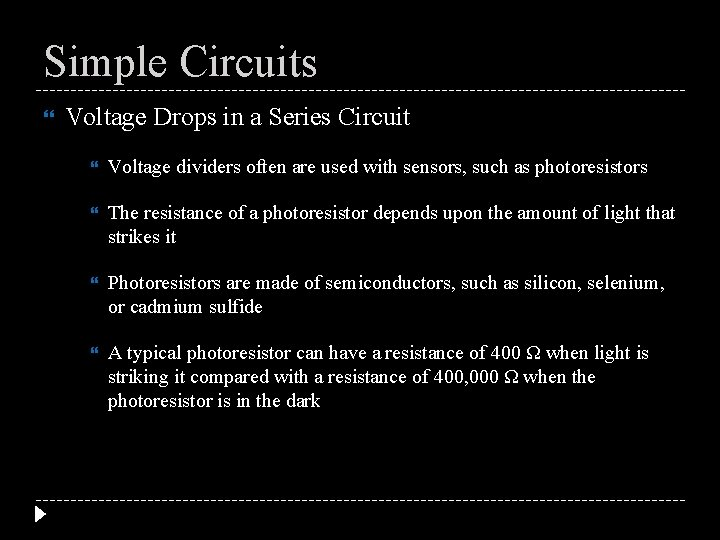Simple Circuits Voltage Drops in a Series Circuit Voltage dividers often are used with sensors, such as photoresistors The resistance of a photoresistor depends upon the amount of light that strikes it Photoresistors are made of semiconductors, such as silicon, selenium, or cadmium sulfide A typical photoresistor can have a resistance of 400 Ω when light is striking it compared with a resistance of 400, 000 Ω when the photoresistor is in the dark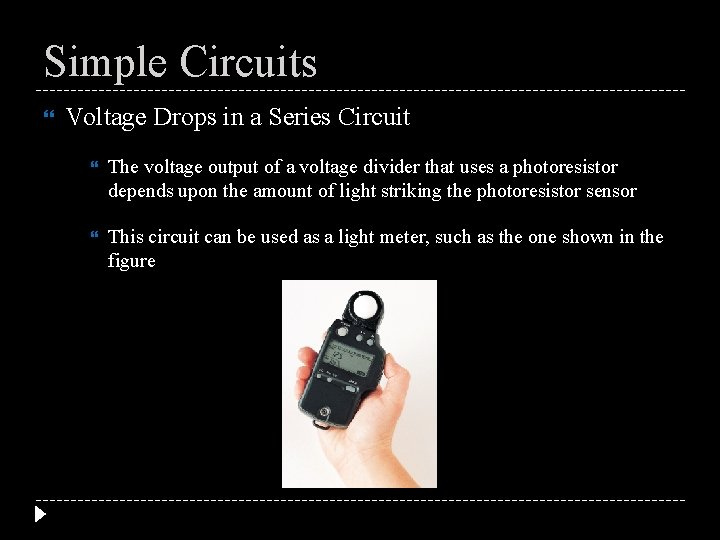Simple Circuits Voltage Drops in a Series Circuit The voltage output of a voltage divider that uses a photoresistor depends upon the amount of light striking the photoresistor sensor This circuit can be used as a light meter, such as the one shown in the figureSimple Circuits Voltage Drops in a Series Circuit In this device, an electronic circuit detects the potential difference and converts it to a measurement of illuminance that can be read on the digital display. The amplified voltmeter reading will drop as illuminance increases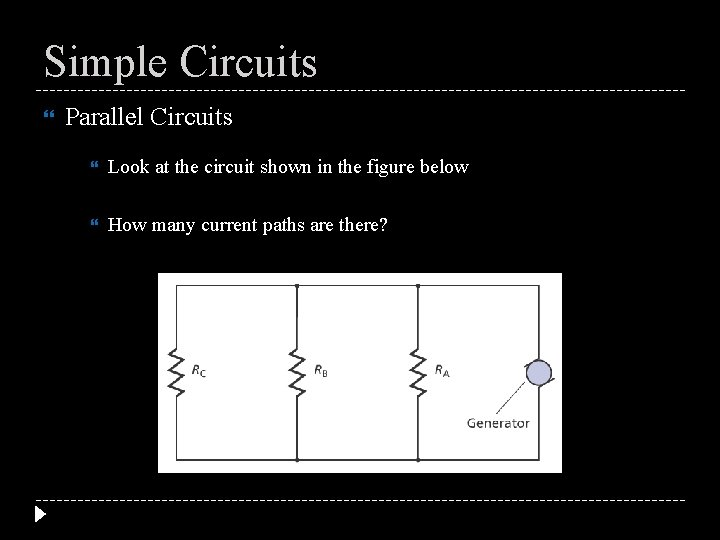Simple Circuits Parallel Circuits Look at the circuit shown in the figure below How many current paths are there?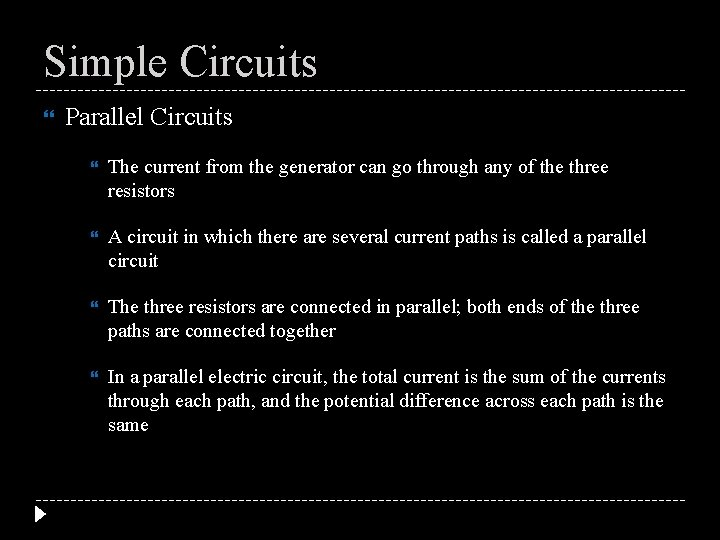Simple Circuits Parallel Circuits The current from the generator can go through any of the three resistors A circuit in which there are several current paths is called a parallel circuit The three resistors are connected in parallel; both ends of the three paths are connected together In a parallel electric circuit, the total current is the sum of the currents through each path, and the potential difference across each path is the sameSimple Circuits Parallel Circuits What is the current through each resistor in a parallel electric circuit? It depends upon the individual resistances For example, in the figure, the potential difference across each resistor is 120 VSimple Circuits Parallel Circuits The current through a resistor is given by I = V/R, so you can calculate the current through the 24 -Ω resistor as I = (120 V)/(24 Ω) = 5. 0 A And then calculate the currents through the other two resistors The total current through the generator is the sum of the currents through the three paths, in this case, 38 A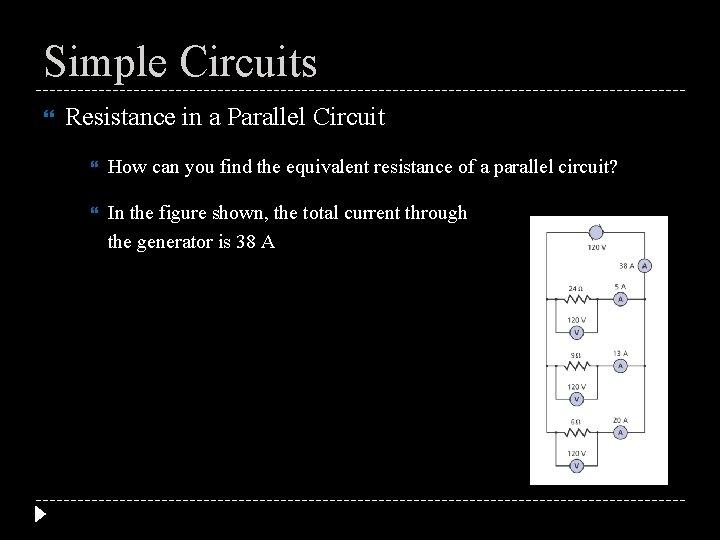Simple Circuits Resistance in a Parallel Circuit How can you find the equivalent resistance of a parallel circuit? In the figure shown, the total current through the generator is 38 A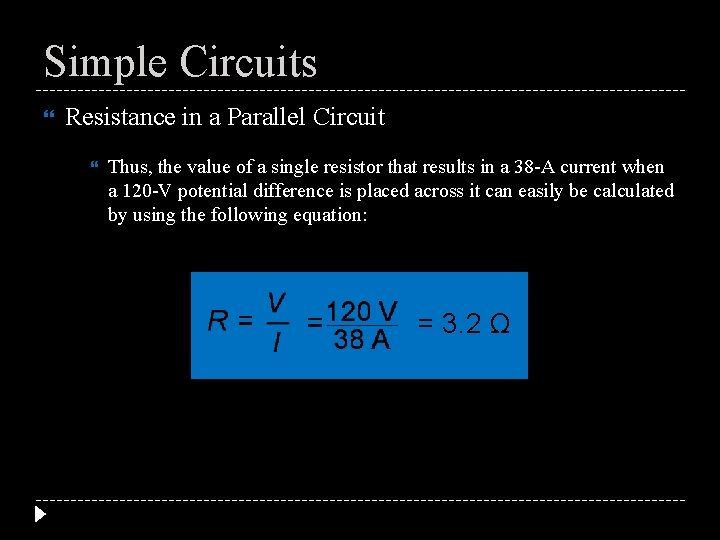Simple Circuits Resistance in a Parallel Circuit Thus, the value of a single resistor that results in a 38 -A current when a 120 -V potential difference is placed across it can easily be calculated by using the following equation: = 3. 2 Ω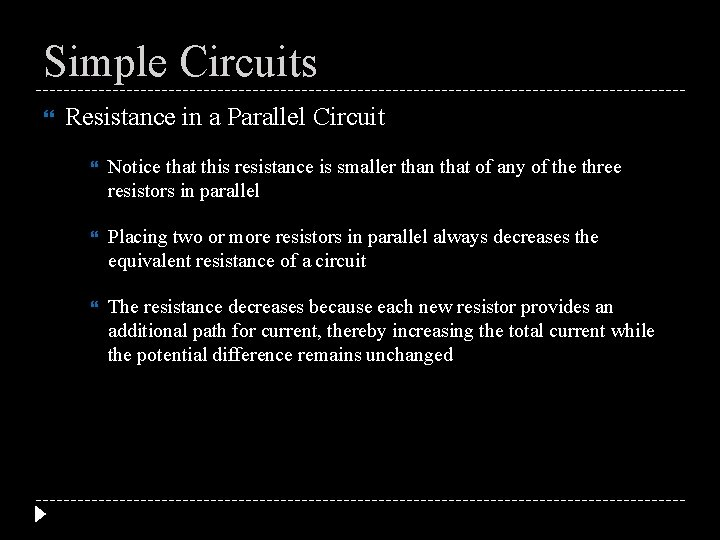Simple Circuits Resistance in a Parallel Circuit Notice that this resistance is smaller than that of any of the three resistors in parallel Placing two or more resistors in parallel always decreases the equivalent resistance of a circuit The resistance decreases because each new resistor provides an additional path for current, thereby increasing the total current while the potential difference remains unchangedSimple Circuits Resistance in a Parallel Circuit To calculate the equivalent resistance of a parallel circuit, first note that the total current is the sum of the currents through the branches If IA, IB, and IC are the currents through the branches and I is the total current, then I = IA + IB + IC The potential difference across each resistor is the same, so the current through each resistor, for example, RA, can be found from IA = V/RA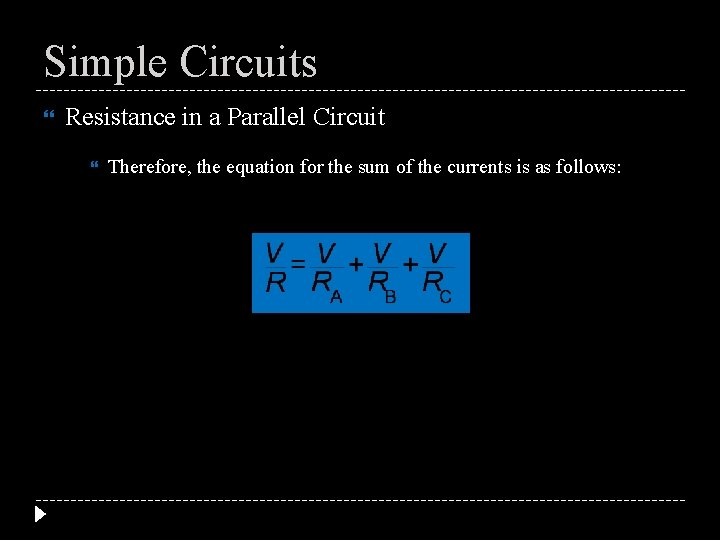Simple Circuits Resistance in a Parallel Circuit Therefore, the equation for the sum of the currents is as follows: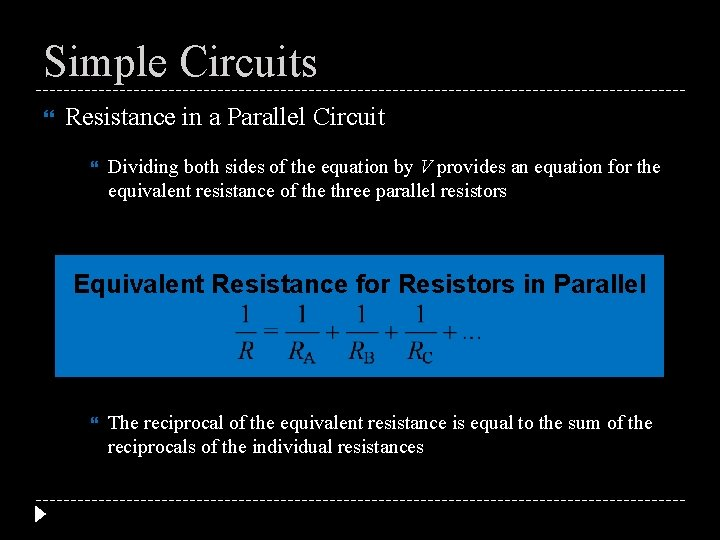Simple Circuits Resistance in a Parallel Circuit Dividing both sides of the equation by V provides an equation for the equivalent resistance of the three parallel resistors Equivalent Resistance for Resistors in Parallel The reciprocal of the equivalent resistance is equal to the sum of the reciprocals of the individual resistances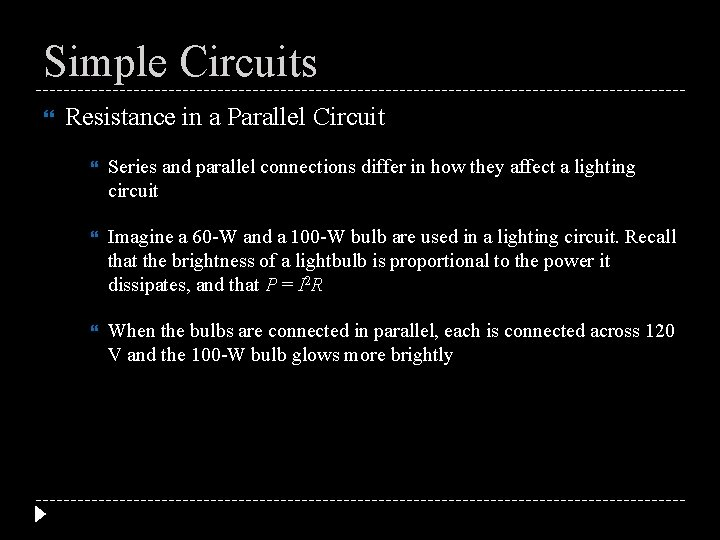Simple Circuits Resistance in a Parallel Circuit Series and parallel connections differ in how they affect a lighting circuit Imagine a 60 -W and a 100 -W bulb are used in a lighting circuit. Recall that the brightness of a lightbulb is proportional to the power it dissipates, and that P = I 2 R When the bulbs are connected in parallel, each is connected across 120 V and the 100 -W bulb glows more brightly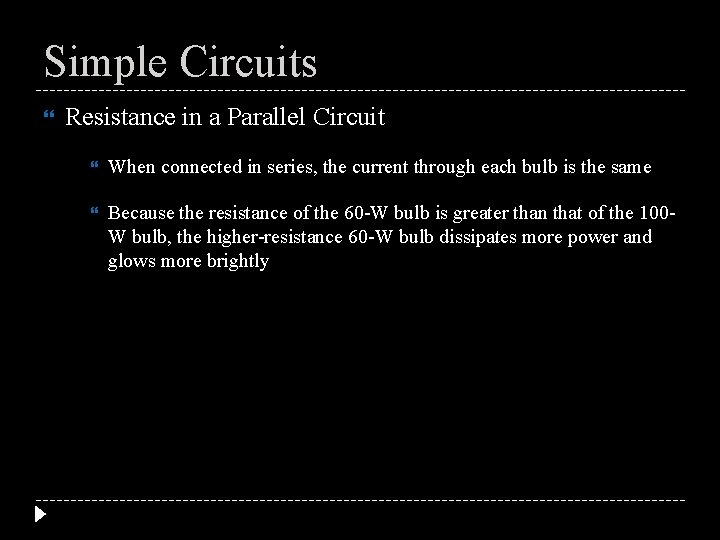Simple Circuits Resistance in a Parallel Circuit When connected in series, the current through each bulb is the same Because the resistance of the 60 -W bulb is greater than that of the 100 W bulb, the higher-resistance 60 -W bulb dissipates more power and glows more brightly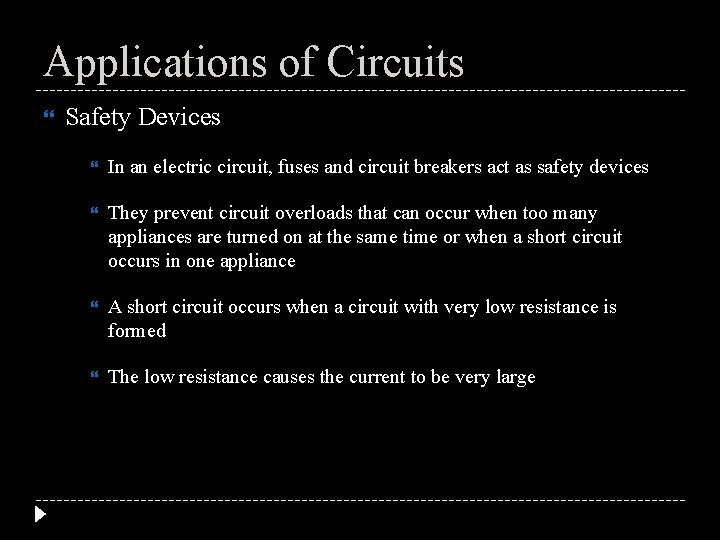Applications of Circuits Safety Devices In an electric circuit, fuses and circuit breakers act as safety devices They prevent circuit overloads that can occur when too many appliances are turned on at the same time or when a short circuit occurs in one appliance A short circuit occurs when a circuit with very low resistance is formed The low resistance causes the current to be very largeApplications of Circuits Safety Devices A fuse is a short piece of metal that melts when too large a current passes through it The thickness of the metal used in a fuse is determined by the amount of current that the circuit is designed to handle safely If a large, unsafe current passes through the circuit, the fuse melts and breaks the circuitApplications of Circuits Safety Devices A circuit breaker is an automatic switch that opens when the current reaches a threshold value If there is a current greater than the rated (threshold) value in the circuit, the circuit becomes overloaded The circuit breaker opens and stops the currentApplications of Circuits Safety Devices A ground-fault interrupter in an electric outlet prevents such injuries because it contains an electronic circuit that detects small differences in current caused by an extra current path and opens the circuit Electric codes for buildings often require ground-fault interrupters to be used in bathrooms, kitchens, and exterior outletsApplications of Circuits Combined Series-Parallel Circuits Have you ever noticed the light in your bathroom or bedroom dim when you turned on a hair dryer? The light and the hair dryer are connected in parallel across 120 V Because of the parallel connection, the current through the light should not have changed when you turned on the hair dryer Yet the light did dim, so the current must have changed The dimming occurred because the house wiring had a small resistance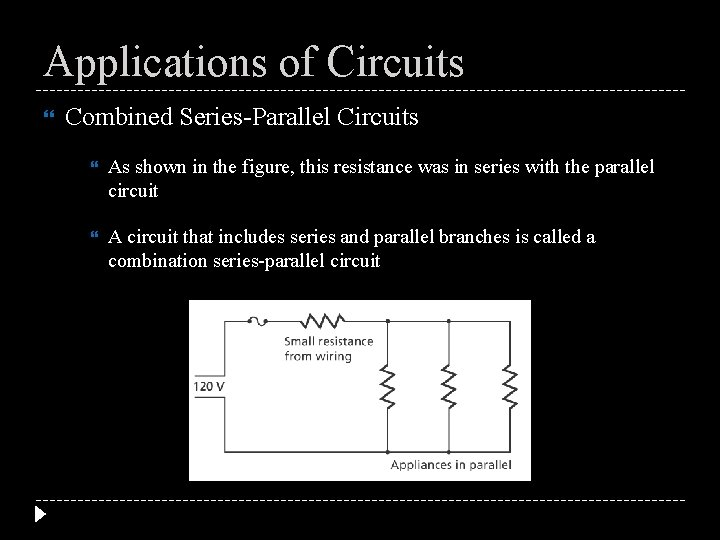Applications of Circuits Combined Series-Parallel Circuits As shown in the figure, this resistance was in series with the parallel circuit A circuit that includes series and parallel branches is called a combination series-parallel circuit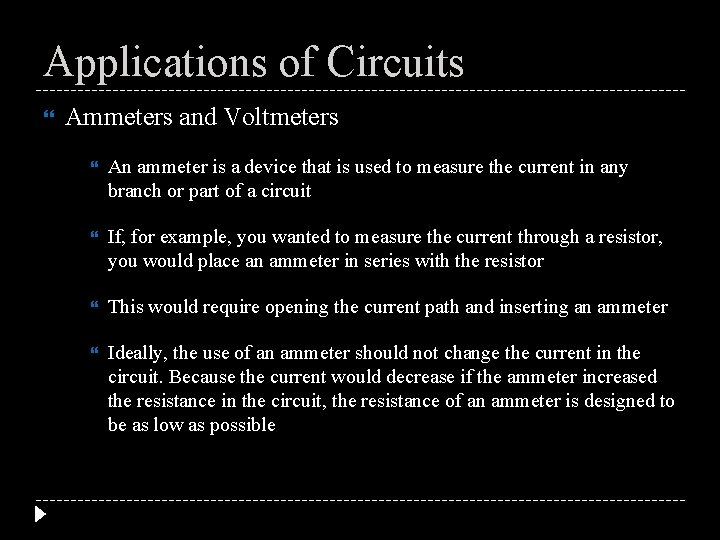Applications of Circuits Ammeters and Voltmeters An ammeter is a device that is used to measure the current in any branch or part of a circuit If, for example, you wanted to measure the current through a resistor, you would place an ammeter in series with the resistor This would require opening the current path and inserting an ammeter Ideally, the use of an ammeter should not change the current in the circuit. Because the current would decrease if the ammeter increased the resistance in the circuit, the resistance of an ammeter is designed to be as low as possible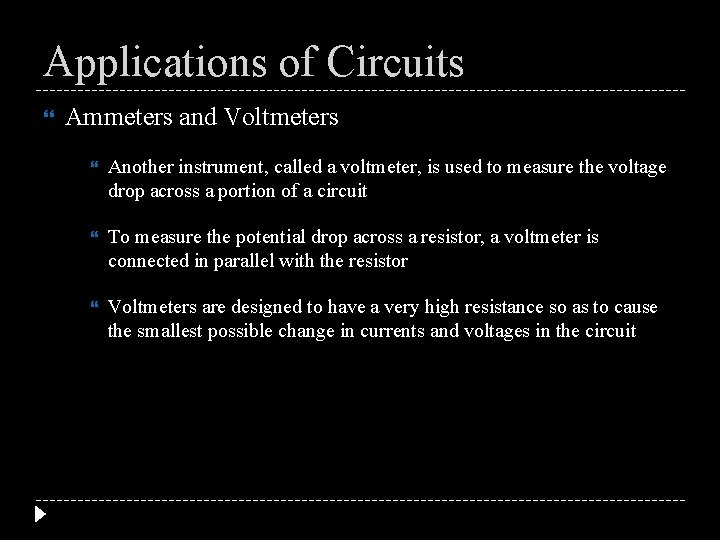Applications of Circuits Ammeters and Voltmeters Another instrument, called a voltmeter, is used to measure the voltage drop across a portion of a circuit To measure the potential drop across a resistor, a voltmeter is connected in parallel with the resistor Voltmeters are designed to have a very high resistance so as to cause the smallest possible change in currents and voltages in the circuitSimple Circuits Three 12. 0 Ω resistors are connected in series to a 50. 0 V power source. What is the equivalent resistance of the circuit? What is the current in the circuit? What is the voltage drop across each? Three 15. 0 Ω resistors are connected in parallel to a 45. 0 V power source. What is the equivalent resistance of the circuit? What is the current in the circuit? What is the current through each resistor? Two resistors are connected in parallel to a 3. 0 V power source. The first resistor is marked as 150 Ω but the second is unmarked. Using an ammeter, it is measured that the current passing through the unknown resistor as 45. 0 m. A. What is the value of the second resistor? What is the current passing through the 150 Ω resistor? What is the total current passing through this power source?